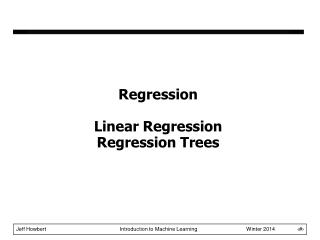DownloadDownload PresentationRegression Linear Regression Regression Trees

# Regression Linear Regression Regression Trees

Télécharger la présentation## Regression Linear Regression Regression Trees

- - - - - - - - - - - - - - - - - - - - - - - - - - - E N D - - - - - - - - - - - - - - - - - - - - - - - - - - -
##### Presentation Transcript

1. RegressionLinear RegressionRegression Trees

2. Characteristics of classification models

3. Loss function • Suppose target labels come from set Y • Binary classification: Y = { 0, 1 } • Regression: Y =  (real numbers) • A loss function maps decisions to costs: • defines the penalty for predicting when the true value is . • Standard choice for classification: 0/1 loss (same as misclassification error) • Standard choice for regression: squared loss

4. Least squares linear fit to data • Most popular estimation method is least squares: • Determine linear coefficients wthat minimize sum of squared loss (SSL). • Use standard (multivariate) differential calculus: • differentiate SSL with respect to w • find zeros of each partial differential equation • solve for each wi • In one dimension:

5. Least squares linear fit to data • Multiple dimensions • To simplify notation and derivation, add a new feature x0 = 1 to feature vector x: • Calculate SSL and determine w:

6. Least squares linear fit to data

7. Least squares linear fit to data

8. Extending application of linear regression • The inputs X for linear regression can be: • Original quantitative inputs • Transformation of quantitative inputs, e.g. log, exp, square root, square, etc. • Polynomial transformation • example: y = w0+ w1x + w2x2 + w3x3 • Basis expansions • Dummy coding of categorical inputs • Interactions between variables • example: x3 = x1 x2 • This allows use of linear regression techniques to fit much more complicated non-linear datasets.

9. Example of fitting polynomial curve with linear model

10. Prostate cancer dataset • 97 samples, partitioned into: • 67 training samples • 30 test samples • Eight predictors (features): • 6 continuous (4 log transforms) • 1 binary • 1 ordinal • Continuous outcome variable: • lpsa: log( prostate specific antigen level )

11. Correlations of predictors in prostate cancer dataset lcavol log cancer volume lweight log prostate weight ageage lbph log amount of benign prostatic hypertrophy svi seminal vesicle invasion lcp log capsular penetration gleasonGleason score pgg45 percent of Gleason scores 4 or 5

12. Fit of linear model to prostate cancer dataset

13. Regularization • Complex models (lots of parameters) often prone to overfitting. • Overfitting can be reduced by imposing a constraint on the overall magnitude of the parameters. • Two common types of regularization (shrinkage) in linear regression: • L2 regularization (a.k.a. ridge regression). Find wwhich minimizes: •  is the regularization parameter: bigger  imposes more constraint • L1 regularization (a.k.a. lasso). Find wwhich minimizes:

14. Example of L2 regularization L2 regularization shrinks coefficients towards (but not to) zero, and towards each other.

15. Example of L1 regularization L1 regularization shrinks coefficients to zero at different rates; different values of  give models with different subsets of features.

16. Example of subset selection

17. Comparison of various selection and shrinkage methods

18. L1 regularization gives sparse models, L2 does not

19. Other types of regression • In addition to linear regression, there are: • many types of non-linear regression • regression trees • nearest neighbor • neural networks • support vector machines • locally linear regression • etc.

20. Regression trees • Model very similar to classification trees • Structure: binary splits on single attributes • Prediction: mean value of training samples in leaf • Induction: • greedy • loss function: sum of squared loss

21. Regression trees

22. Regression tree loss function • Assume: • Attribute and split threshold for candidate split are selected • Candidate split partitions samples at parent node into child node sample sets C1 and C2 • Loss for the candidate split is:

23. Characteristics of regression models

24. MATLAB interlude matlab_demo_08.m